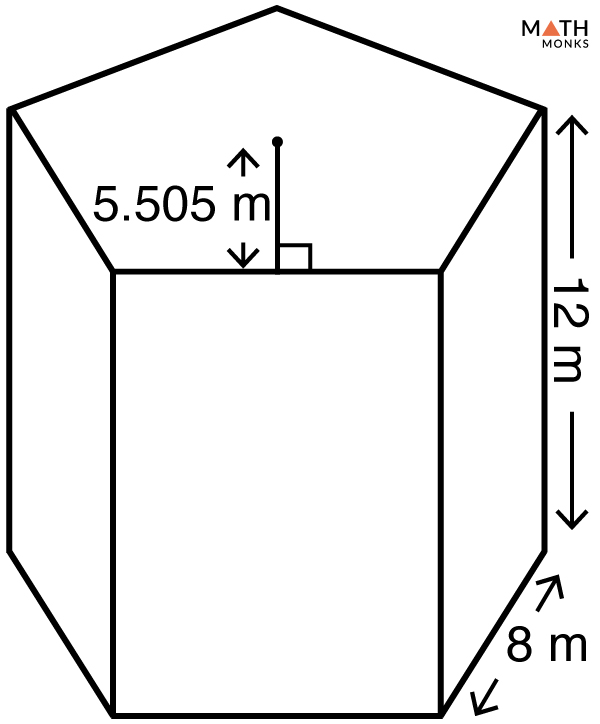# Surface Area of a Pentagonal Prism

The surface area (or total surface area) of a pentagonal prism is the entire amount of space occupied by all its outer surfaces (or faces). It is measured in square units such as m2, cm2, mm2, and in2.

## Formula

The formula is given below:

Let us solve some examples to understand the concept better.

## Solved ExamplesFind the lateral and the total surface area of a pentagonal prism given in the figure.

Solution:

As we know,
Lateral Surface Area (LSA) = 5bh, here b = 8 m, h = 12 m
LSA = 5 × 8× 12
= 480 m2
Total Surface Area (TSA) = 5ab + LSA, here a = 5.505 m, LSA = 480
TSA = 5 × 5.505 × 8 + 480
= 700.2 m2

Find the lateral area of a pentagonal prism with a base edge of 5.5 cm and height of 11 cm.

Solution:

As we know,
Lateral Surface Area (LSA) = 5bh, here b = 5.5 cm, h = 11 cm
LSA = 5 × 5.5 × 11
= 302.5 cm2

Finding the surface area of a pentagonal prism when the BASE EDGE and HEIGHT are knownFind the surface area of a pentagonal prism with a base edge of 11 cm and height 6 cm.

Solution:

As we know,
Total Surface Area (TSA) = ${ 5bh+\dfrac{1}{2}\sqrt{5\left( 5+2\sqrt{5}\right) }b^{2} }$ , here  b = 11 cm, h = 6 cm
∴ TSA = ${ 5\times 11\times 7+\dfrac{1}{2}\sqrt{5\left( 5+2\sqrt{5}\right) }\times 11^{2}}$
= 801.35 cm2

• More Resources: